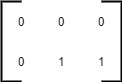Problem title
Difficulty
Avg time to solve

SQL Practice
Easy
--
Longest Substring Without Repeating Characters
Moderate
30 mins
Tiling Problem
Hard
45 mins
Fahrenheit to Celsius
Easy
15 mins
Detect And Remove Cycle
Easy
10 mins
Matrix Chain Multiplication
Moderate
40 mins
Multiply Strings
Moderate
35 mins
Quick Sort
Moderate
10 mins
Reverse List In K Groups
Moderate
15 mins
Course Schedule
Easy
15 mins23

# Leftmost Column With At Least A One

Difficulty: MEDIUM
Avg. time to solve
30 min
Success Rate
70%

Problem Statement

#### However, you can not access the binary matrix directly. The function dimensions() return an array of two elements [m, n] which denote the number of rows and the number of columns in the matrix respectively. The function get(x, y) returns the element of the matrix at the index (x, y).

##### Note :
``````We are following 0-based indexing for this problem. The get method should be called for a max of 2000 times, and the dimensions method should be called only once. Submissions making more than 2000 calls to the get method and more than one call to the dimensions will be judged as the wrong answers.
``````
##### For Example :
``````If the BinaryMatrix has 2 rows and 3 columns and the matrix is {{0, 0, 0}, {0, 1, 1}}. Then, both the second and the third columns contain a 1, but the second column is the leftmost column. Hence, the answer is 1 (0-based indexing).
````````````Note that, here, the BinaryMatrix is given as an example. In the actual problem, you have to access the elements of the matrix by using the BinaryMatrix.get() method.
``````
##### Input format :
``````The first line contains an integer 'T', which denotes the number of test cases or queries to be run. Then, the T test cases follow.

The first line of each test case contains two space-separated integers M and N, denoting the number of rows and the number of columns in the matrix, respectively.

Then M lines follow. Each line contains N space-separated integers denoting elements of the matrix.

Note that the input is given for reference. You can’t access the elements of the matrix directly. You have to use the get method to do so.
``````
##### Output format :
``````For each test case, print the leftmost index (0-based indexing) of the column such that it contains at least one 1. If such a column doesn’t exist in the matrix, then return -1.

Output for each test case will be printed in a separate line.

If the program calls the get method more than 2000 times or the dimensions function more than once, then it will be judged as a wrong answer, and the program stops instantly.
``````
##### Note :
``````You do not need to print anything. It has already been taken care of. Just implement the given function.
``````
##### Constraints :
``````1 <= T <= 5
1 <= M <= 1000
1 <= N <= 1000
0 <= BinaryMatrix[i][j] <= 1

Time limit: 1 second
``````
##### Sample Input 1 :
``````2
2 2
0 0
1 1
3 2
0 0
0 0
0 0
``````
##### Sample Output 1 :
``````0
-1
``````
##### Explanation for sample 1 :
``````For the first test case, the first and second columns both have element one present. So, the leftmost column is the first column. Hence, the answer is 0 (0-based indexing).

For the second test case, there is no one present in the matrix. Hence, we print -1.
``````
##### Sample Input 2 :
``````2
1 1
1
3 3
0 0 0
0 0 0
0 0 1
``````
##### Sample Output 2 :
``````0
2
``````Console